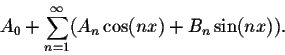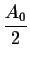#Introduction to Fourier SeriesOn a previous page, we have discussed how Taylor polynomials approximate functions. The only problem with these polynomials is the fact that the approximation is done locally not globally. So if you are interested to find a limit at a point then Taylor approximations are good. But if you desire to approximate an integral over an interval, then Taylor approximations fail.
J.B. Fourier (1768-1830) was among the first to investigate this problem. In his book Théorie Analytique de la Chaleur'', written in 1822, he introduced the concept of Fourier series which he used extensively. Recall that a Fourier series is any expression of the formSome authors useinstead A0.[Geometry] [Algebra] [Trigonometry ]
[Calculus] [Differential Equations] [Matrix Algebra]S.O.S MATH: Home Page

Do you need more help? Please post your question on our S.O.S. Mathematics CyberBoard.Author: M.A. Khamsi